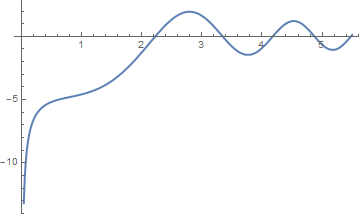# Preface

This tutorial was made solely for the purpose of education and it was designed for students taking Applied Math 0330. It is primarily for students who have very little experience or have never used Mathematica and programming before and would like to learn more of the basics for this computer algebra system. As a friendly reminder, don't forget to clear variables in use and/or the kernel. The Mathematica commands in this tutorial are all written in bold black font, while Mathematica output is in normal font.

Finally, you can copy and paste all commands into your Mathematica notebook, change the parameters, and run them because the tutorial is under the terms of the GNU General Public License (GPL). You, as the user, are free to use the scripts for your needs to learn the Mathematica program, and have the right to distribute this tutorial and refer to this tutorial as long as this tutorial is accredited appropriately. The tutorial accompanies the textbook Applied Differential Equations. The Primary Course by Vladimir Dobrushkin, CRC Press, 2015; http://www.crcpress.com/product/isbn/9781439851043

# Milne--Simpson Method

Another popular predictor-corrector scheme is known as the Milne or Milne--Simpson method. See
Milne, W. E., Numerical Solutions of Differential Equations, Wiley, New York, 1953.
Its predictor is based on integration of the slope function f(t, y(t)) over the interval $$\left[ x_{n-3} , x_{n+1} \right]$$ and then applying the Simpson rule:

$y(x_{n+1}) = y(x_{n-3}) + \int_{x_{n-3}}^{x_{n+1}} f(t, y(t)) \,{\text d}t .$
The predictor uses the Lagrange polynomial approximation for f(t, y(t)) based on four mesh points $$(x_{n-3} , f_{n-3} ), \ (x_{n-2} , f_{n-2} ), \ (x_{n-1} , f_{n-1} ), \ (x_{n} , f_{n} ).$$ It is integrated over the interval $$\left[ x_{n-3} , x_{n+1} \right] .$$ This produces the Milne predictor:
$p_{n+1} = y_{n-3} + \frac{4h}{3} \left( 2\,f_{n-2} - f_{n-1} + 2\,f_n \right) , \qquad n=3,4,\ldots .$
The above formula was discovered by William Edmund Milne in 1953. This formula has local truncation error of order O(h5). The Milne corrector is developed similarly.
$y_{n+1} = y_{n-1} + \frac{h}{3} \left( f_{n-1} +4\, f_{n} + f_{n+1} \right) , \qquad n=3,4,\ldots ;$
where $$f_{n+1} = f \left( x_{n+1} , p_{n+1} \right)$$ is based on the predictor value pn+1. The Milne--Simpson multistep method is sometimes unstable. The Milne method converges when
$h < \frac{3}{\left\vert f_y (x_n , y_n ) \right\vert} , \qquad n=0,1,2,\ldots .$

Example. Let us start with the Riccati equation $$y' = x^2 + y^2$$ subject to the initial condition $$y(0) =-1 .$$ Its solution is expressed through Bessel functions:

$d(x)\, y(x) = x \left[ J_{-3/4} \left( \frac{x^2}{2} \right) \left( \Gamma^2 \left( \frac{3}{4} \right) + \pi \right) - Y_{-3/4} \left( \frac{x^2}{2} \right) \Gamma^2 \left( \frac{3}{4} \right) \right] .$
where the denominator
$d(x) = Y_{1/4} \left( \frac{x^2}{2} \right) \Gamma^2 \left( \frac{3}{4} \right) - \left[ J_{1/4} \left( \frac{x^2}{2} \right) \left( \Gamma^2 \left( \frac{3}{4} \right) +\pi \right) \right]$
has the first positive null at x = 2.223378383 as the figure shows
d[x_] = BesselY[1/4, x^2 /2]*Gamma[3/4]^2 - BesselJ[1/4, x^2 /2]*(Gamma[3/4]^2 + Pi)
Plot[d[x], {x, 0, 5.5}, PlotStyle -> Thick]The sollution blows up near the point 2.223378.